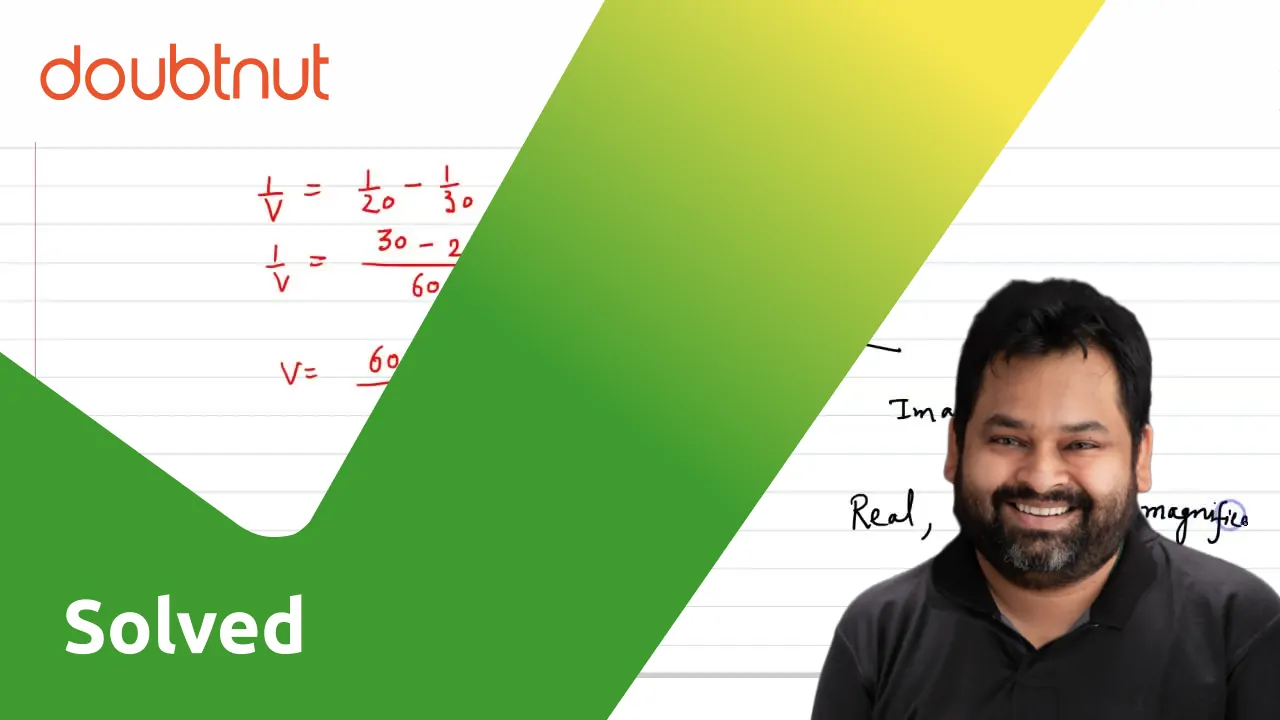Doubtnut App - Get 50 Lakh+ video solutions better than GoogleInstall Now

# An object is placed at a distance of 30 cm from a convex lens of focal length 20 cm.

Text Solution
Verified by Experts

## In the given problem Object distance, u = - 30 cm (from sign convention) Image distance, v = ? Focal length of the lens f = 20 cm From lens formula 1f=1v−1u 120=1v−1−30 120=1v+130 1v=120−130 1v=30−2030×20=10600 v=60010=60 cm . Magnification, m = height of the imageheight of the object =vu M=60−30=−2. Negative sign indicates that the image is inverted . Since, m is greater than one, the image is magnified. Since v is positive, the image is real. The image is formed 60 cm on the other side of the lens and it is real, inverted and magnified.